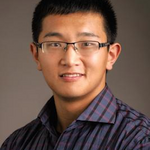Community Profile# Jin Yang

### The University of Texas at Austin

Last seen: 1 day ago Active since 2020

Assistant professor at the UT-Austin Aerospace Engineering and Engineering Mechanics department. Previously: Postdoc at the University of Wisconsin-Madison; Ph.D., Caltech, 2019; B.En., Tsinghua University, 2013.

#### Statistics

All
•••••••••#### Content Feed

View by

Solved

The Piggy Bank Problem
Given a cylindrical piggy bank with radius g and height y, return the bank's volume. [ g is first input argument.] Bonus though...

12 months ago

Solved

Prime factor digits
Consider the following number system. Calculate the prime factorization for each number n, then represent the prime factors in a...

2 years ago

Solved

Calculate Amount of Cake Frosting
Given two input variables |r| and |h|, which stand for the radius and height of a cake, calculate the surface area of the cake y...

2 years ago

Solved

Times 2 - START HERE
Try out this test problem first. Given the variable x as your input, multiply it by two and put the result in y. Examples:...

2 years ago